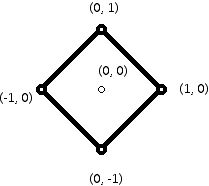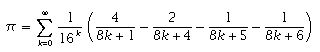Prepared by:
Joseph Malkevitch
Department of Mathematics and Computing
York College (CUNY)
Jamaica, New York 11451

Email: malkevitch@york.cuny.edu (for additions, suggestions, and corrections)

web page: http://www.york.cuny.edu/~malk

Pi, or π, as it is universally denoted, is a remarkable source of mathematical arcania.

What is π?

π is defined to be the ratio of the circumference of a (Euclidean) circle to the diameter of the circle. Amazingly, this number is a constant independent of the radius of the circle. The reason one should explicitly mention Euclidean circle is that one can have circles using other distance functions where one represents points by ordered pairs of real numbers. Thus, in the taxicab plane, the distance between the points (x1, x2) and (y1, y2) is given by |x1-x2| + |y1 - y2|. The taxicab circle of radius 1 about (0,0) is shown below. It is easy to verify that for any taxicab circle the ratio of the "circumference" to the diameter is 4! (In the example below, the circumference is 8 because each side of the circle has length 2, and the diameter of the circle is 2. Thus, "pi" for the taxicab plane is 4.)Remarkably, in ancient times (Egypt, Greece, China, and India) information about pi was already known. Archimedes (287-212 BC) had already estimated the size of pi based on using inscribed and circumscribed polygons about a circle.

π is irrational

A number is rational if it can be written in the form a/b where a and b are integers and b is not zero. π is not a rational number though it is somewhat difficult to show this. Thus, to represent π in the decimal system one needs to use an infinite non-repeating decimal. (The only terminating and repeating decimals arise from rational numbers.) However, it is nonetheless of interest to give "good" rational approximations to π. The most famous of these is 22/7 which was already known in ancient times. However, there is a surprisingly good rational representation of pi that uses only 6 digits: 355/113. This rational number has the following starting digits (remember it is a repeating decimal): 3.1415929203539825. The start digits for the exact value of pi is given by 3.14159 26535 89793.

We know pi is irrational because Johann Lambert (1728-1777) gave a proof of this fact. Lambert's approach, thought by some not to meet modern standards of rigor, seems, in fact, notable for being a complex result which was proved in a way to meet modern standards though the work was done in the 18th century. (Lambert is also famous for his work related to the development of Non-Euclidean Geometry.)

Pi is not only irrational, it is also transcendental. A number is transcendental if it is not the root of an algebraical (polynomial) equation with integer coefficients. In 1882, Ferdinand Lindemann (1852-1939) showed that pi was transcendental. (Lindemann was a student of Felix Klein (1849-1925) and he, in turn, was the doctoral supervisor of David Hilbert (1862-1943)).

The digits of pi

Why should one be interested in calculating pi to more and more decimal places? Clearly, it is of no use to a carpenter or plumber to have pi computed to many millions of decimal places. However, beyond the desire to have a spot in a "record book" for having computed accurately the largest number of digits in a decimal representation of pi, there are practical reasons for doing this! For example, modern computer chips are getting more and more complicated to design. Some years ago it was discovered that Intel was producing a flawed chip. The flaw was subtle and only showed up in particular types of calculations. It has been suggested that one way to test chips for design flaws is to use new chips for complex calculations such as those involved in generating millions of digits for pi.

Although there are a variety of algorithms (many of them based on infinite series or infinite products) that will compute pi in principle to any number of digits that one might desire, the usual algorithms for doing this required that to compute, say, the 1,000,000th digit one would first have to have computed the 999,999th digit. In 1995, David Bailey, Peter Borwein and Simon Plouffe discovered a new and relatively simple infinite series for pi:Based on this formula and extensions of it, it was discovered how to compute the kth digit of pi in base b without having to compute all of the earlier digits in pi's base b expansion. These formulas have come to be known as BBP-formulas and they have proved useful for computing other transcendental numbers in addition to pi.

1. In the decimal representation of pi does each of the digits 0, 1, ..., 9 appear infinitely often?

2. As the number of digits in the decimal representation of pi gets larger and larger, does the number of occurrences of each digit get closer and closer to 1/10?

3. For every decimal digit k, is there somewhere in the decimal expansion of pi where a block of m consecutive occurrences of k occur?

References:

Adamchik, V. and Wagon, S. "A Simple Formula for pi." Amer. Math. Monthly 104 (1997) 852-855.

Bailey, D. H. and Borwein, J. M. "Experimental Mathematics: Examples, Methods, and Implications." Notices Amer. Math. Soc. 52 (2005) 502-514.

Beckmann, P., A history of π, St. Martins Press, New York, 1976.

Kulkarni, R., The value of π known to Sulbasutrakaras, Indian J. Hist. Sci. 13 (1) (1978), 32-41.

Lambert, A.M. Irrationality of pi, in A Source Book in Mathematics 1200-1800, Princeton University Press, Princeton, 1986, pp. 369 - 374

Volkov, A., Calculation of π in ancient China : from Liu Hui to Zu Chongzhi, Historia Sci. (2) 4 (2) (1994), 139-157.

Roy, R., The discovery of the series formula for π by Leibniz, Gregory and Nilakantha, Math. Mag. 63 (1990) 291-306.

Zembrowski, E., A History of the Circle, Rutgers University Press, New Brunswick, 1999.

Web resources

There are many excellent places to learn about pi on the web:

A few good places to get started are:

Mathworld (http://mathworld.wolfram.com/Pi.html)

Formulas (http://mathworld.wolfram.com/PiFormulas.html)

Wikipedia (http://en.wikipedia.org/wiki/Pi)

Trivia and a lot more (http://www.cecm.sfu.ca/~jborwein/pi_cover.html)

History

(http://wwwgroups.dcs.stand.ac.uk/~history/HistTopics/Pi_through_the_ages.html)

Many of these sources are good places for learning a lot more mathematics beyond learning about pi.

Acknowledgment

This work was supported in part by the Teacher Academy of York College. Specific funding was provided by: FIPSE (46274-07 01) and the Fund for PS (72042-07 01) to the Teacher Academy of CUNY.